This video will show
• Making sure it’s converging
• Get the right learning rate, alpha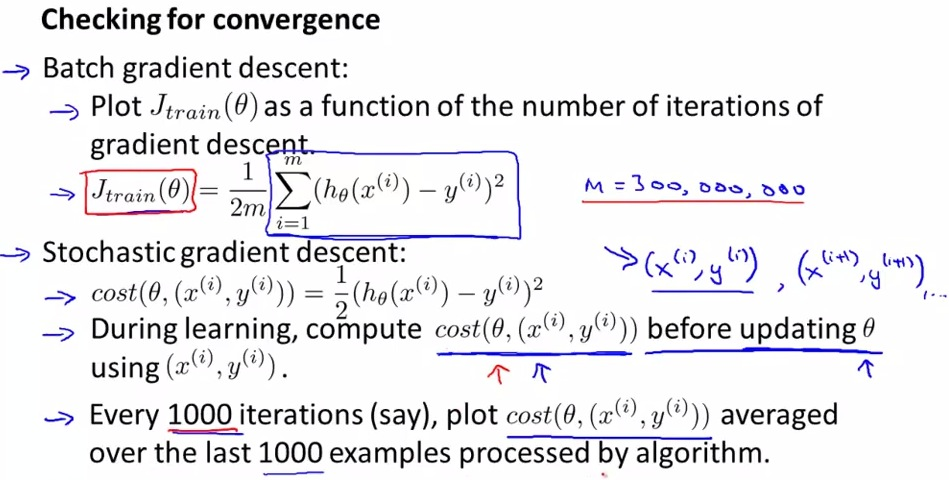• As talked earlier, batch gradient descent wait for particular huge amount of time before updating.
• In stochastic, to make sure its converging, compute the cost before updating thetas, and plot the cost function average last 1000 examples.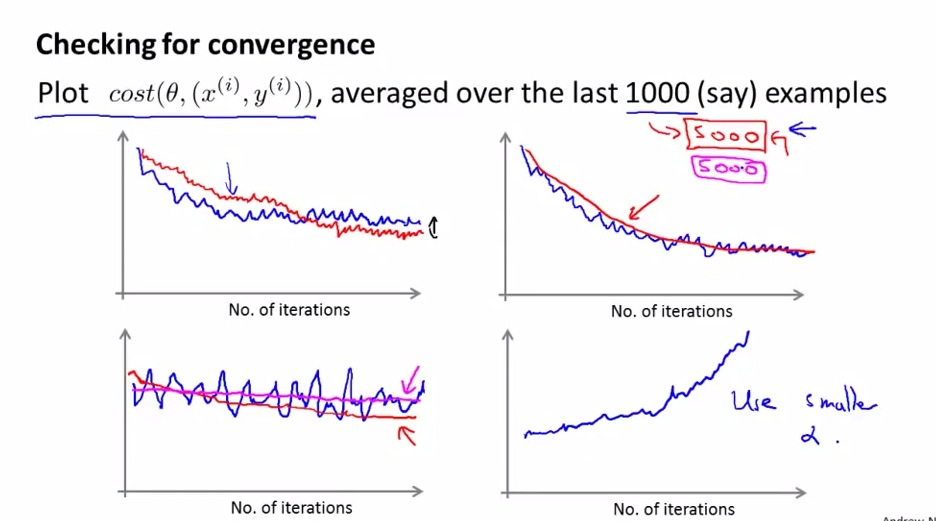• These are 4 different plots that we might encounter when using stochastic GD and plot with 1000 examples
1. Good Stochastic
• In this case the up and down(stochastic) shown and see a point where the actual minimum of cost function
• In red line, when we choose smaller alpha, we see that it actually show better the minimum cost function
2. Second
• The second shown in blue line with 1000 examples, still stochastic
• When using 5000 examples we actually see smoother line as shown in red, but the drawback is we get delayed feedback of learning rate
3. Third
• Here’s we actually shown that the algorithm may not learning at all
• The red shown as we see that the trend may actually converging down to minimum, we just didn’t see it because it’s too stochastic with blue(1000 examples)
• Of course it may also be that we have a flat line, this case try to use more features
4. Fourth
The algorithm is diverging, vastly overshoot. In this case we try to use smaller alpha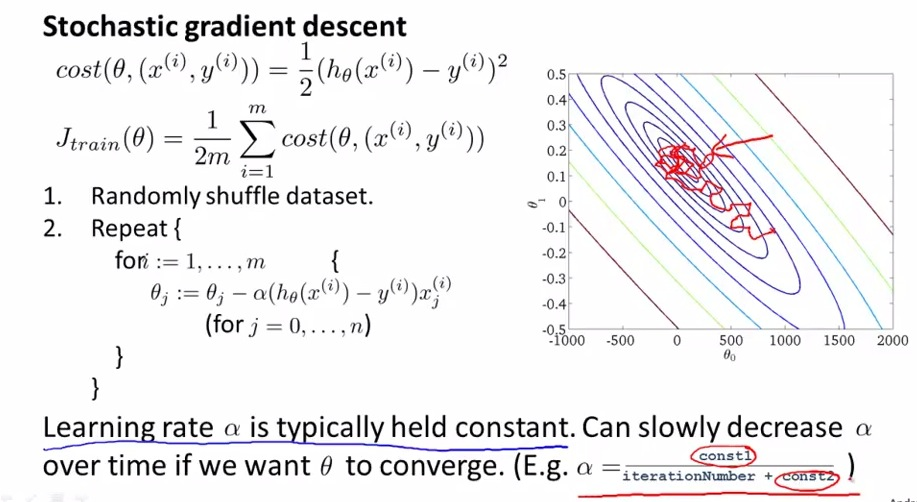• So the other way, it’s also important think about the learning rate alpha
• Above diagram is when we using learning rate constant, whereas below when we keep setting the learning rate smaller and smaller
• The way we set learning rate smaller is by taking 3 value, iterNumber, cons1 and const2
• iterNumber keep increasing as we move iteration forward, that way alpha is getting smaller
• Where cons1 and cons2 is a constant number that we play a bit to find the correct ones.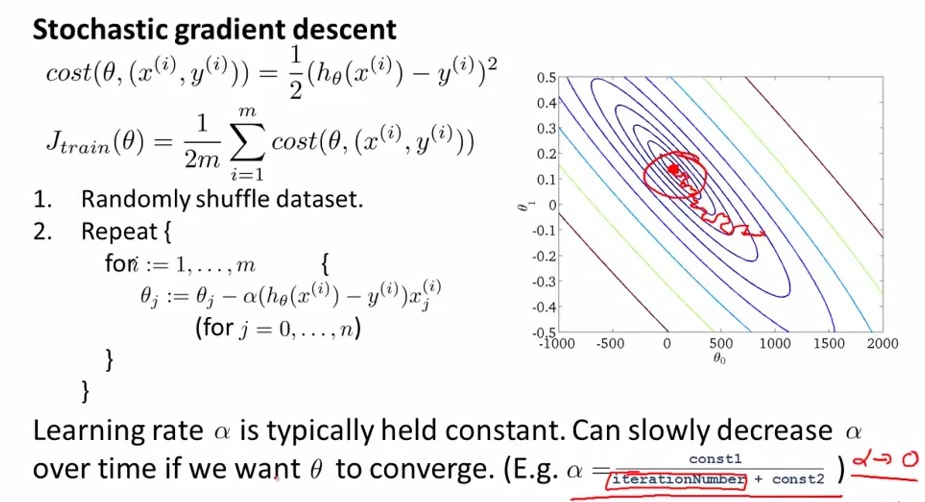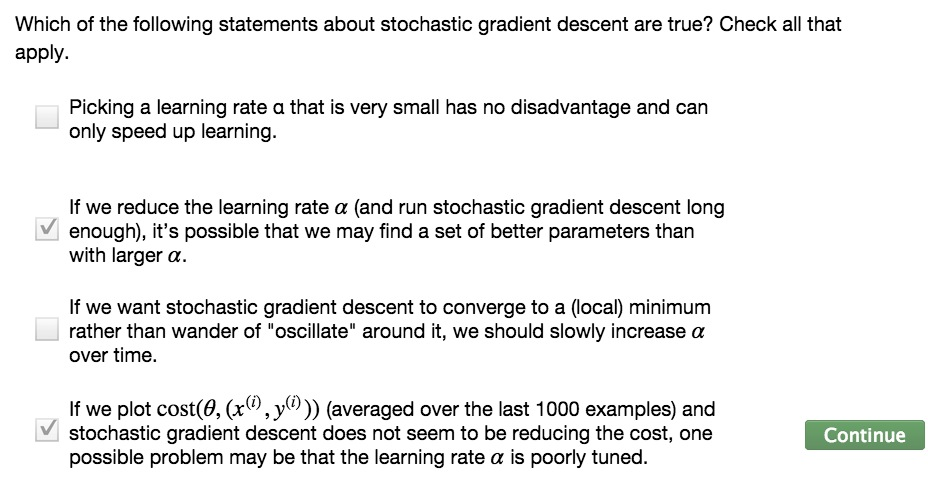• So there are two ways to making sure the stochastic is converging
1. Plot the cost function to make sure it’s converging
2. Slowly make smaller alpha
some scientist just using the alpha constant, where some other scientist set the alpha to keep getting smaller.